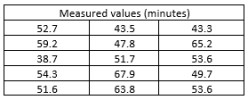Probability & Statistics

•0

Calculating Probabilities for Normal and Non-Normal Distributions

17 months ago

We show different ways to calculate probabilities. The first example illustrates a case where the data sample is normal and the second example for a case where the sample distribution is not normal. It is used three tools: Excel, Minitab, Universal Probability Calculator

•2

Do You See False Patterns in Random Data?

2 months ago

Coincidence or the presence of a certain third, unseen factor? Is this how a conspiracy theory is born? An illusory correlation or in Latin, 'um hoc ergo propter hoc', "with this, therefore because of this," also called the false cause.

•0

Taking a Chance: How Probability Affects Us Every Day

17 months ago

How does probability influence us in our daily lives? This article takes a brief look at the way we look at problems and the influences on our decisions.

working